JMSLTM Numerical Library 7.2.0
com.imsl.math

## Class Spline2D

• All Implemented Interfaces:
Serializable, Cloneable
Direct Known Subclasses:
Spline2DInterpolate, Spline2DLeastSquares

```public abstract class Spline2D
extends Object
implements Serializable, Cloneable```
Represents and evaluates tensor-product splines.

The simplest method of obtaining multivariate interpolation and approximation functions is to take univariate methods and form a multivariate method via tensor products. In the case of two-dimensional spline interpolation, the derivation proceeds as follows: Let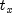be a knot sequence for splines of order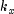, and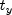be a knot sequence for splines of order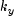. Let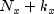be the length of, and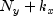be the length of. Then, the tensor-product spline has the following form: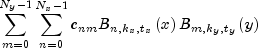Given two sets of points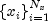and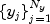for which the corresponding univariate interpolation problem can be solved, the tensor-product interpolation problem finds the coefficients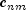so that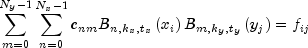This problem can be solved efficiently by repeatedly solving univariate interpolation problems as described in de Boor (1978, p. 347).

Spline2DInterpolate Example, Serialized Form
• ### Constructor Summary

Constructors
Constructor and Description
`Spline2D()`
• ### Method Summary

Methods
Modifier and Type Method and Description
`double[][]` ```derivative(double[] xVec, double[] yVec, int xPartial, int yPartial)```
Returns the values of the partial derivative of the tensor-product spline of an array of points.
`double` ```derivative(double x, double y, int xPartial, int yPartial)```
Returns the value of the partial derivative of the tensor-product spline at the point (x, y).
`double[][]` `getCoefficients()`
Returns the coefficients for the tensor-product spline.
`double[]` `getXKnots()`
Returns the knot sequences in the x-direction.
`double[]` `getYKnots()`
Returns the knot sequences in the y-direction.
`double` ```integral(double a, double b, double c, double d)```
Returns the value of an integral of a tensor-product spline on a rectangular domain.
`double[][]` ```value(double[] xVec, double[] yVec)```
Returns the values of the tensor-product spline of an array of points.
`double` ```value(double x, double y)```
Returns the value of the tensor-product spline at the point (x, y).
• ### Methods inherited from class java.lang.Object

`clone, equals, finalize, getClass, hashCode, notify, notifyAll, toString, wait, wait, wait`
• ### Constructor Detail

• #### Spline2D

`public Spline2D()`
• ### Method Detail

• #### derivative

```public double[][] derivative(double[] xVec,
double[] yVec,
int xPartial,
int yPartial)```
Returns the values of the partial derivative of the tensor-product spline of an array of points.
Parameters:
`xVec` - a `double` array specifying the x-coordinates at which the spline is to be evaluated.
`yVec` - a `double` array specifying the y-coordinates at which the spline is to be evaluated.
`xPartial` - an `int` scalar specifying the x-partial derivative.
`yPartial` - an `int` scalar specifying the y-partial derivative.
Returns:
a `double` matrix containing the values of the partial derivatives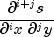where i = `xPartial` and j = `yPartial`, at each (x, y).
• #### derivative

```public double derivative(double x,
double y,
int xPartial,
int yPartial)```
Returns the value of the partial derivative of the tensor-product spline at the point (x, y).
Parameters:
`x` - a `double` scalar specifying the x-coordinate of the evaluation point for the tensor-product spline.
`y` - a `double` scalar specifying the y-coordinate of the evaluation point for the tensor-product spline.
`xPartial` - an `int` scalar specifying the x-partial derivative.
`yPartial` - an `int` scalar specifying the y-partial derivative.
Returns:
a `double` scalar containing the value of the partial derivativewhere i = `xPartial` and j = `yPartial`, at (x, y).
• #### getCoefficients

`public double[][] getCoefficients()`
Returns the coefficients for the tensor-product spline.
Returns:
a `double` matrix containing the coefficients.
• #### getXKnots

`public double[] getXKnots()`
Returns the knot sequences in the x-direction.
Returns:
a `double` array containing the knot sequences of the spline in the x-direction.
• #### getYKnots

`public double[] getYKnots()`
Returns the knot sequences in the y-direction.
Returns:
a `double` array containing the knot sequences of the spline in the y-direction.
• #### integral

```public double integral(double a,
double b,
double c,
double d)```
Returns the value of an integral of a tensor-product spline on a rectangular domain.

If s is the spline, then the `integral` method returns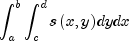This method uses the (univariate integration) identity (22) in de Boor (1978, p. 151)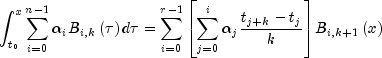where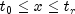.

It assumes (for all knot sequences) that the first and last k knots are stacked, that is,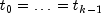and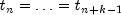, where k is the order of the spline in the x or y direction.

Parameters:
`a` - a `double` specifying the lower limit for the first variable of the tensor-product spline.
`b` - a `double` specifying the upper limit for the first variable of the tensor-product spline.
`c` - a `double` specifying the lower limit for the second variable of the tensor-product spline.
`d` - a `double` specifying the upper limit for the second variable of the tensor-product spline.
Returns:
a `double`, the integral of the tensor-product spline over the rectangle `[a, b]` by `[c, d]`.
• #### value

```public double[][] value(double[] xVec,
double[] yVec)```
Returns the values of the tensor-product spline of an array of points.
Parameters:
`xVec` - a `double` array specifying the x-coordinates at which the spline is to be evaluated.
`yVec` - a `double` array specifying the y-coordinates at which the spline is to be evaluated.
Returns:
a `double` matrix containing the values evaluated.
• #### value

```public double value(double x,
double y)```
Returns the value of the tensor-product spline at the point (x, y).
Parameters:
`x` - a `double` scalar specifying the x-coordinate of the evaluation point for the tensor-product spline.
`y` - a `double` scalar specifying the y-coordinate of the evaluation point for the tensor-product spline.
Returns:
a `double` scalar containing the value of the tensor-product spline.
JMSLTM Numerical Library 7.2.0